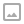## The Change-Making Problem - Exploring The Dynamic Programming Solution

·   ·  ☕ 2 min read

You work at a restaurant on a a foreign planet. Your manager demands that all change be dispensed precisely and that it always be given with the least number of coins possible. How do you do that?

In this video we’ll look at two ways to solve this problem. We’ll begin with the greedy algorithm solution (the wrong solution) which will simply use the highest available coin possible until the full change amount is made. This solution will work for many modern coin amounts such as American currency due to the denominations of the coins. However, it does not provide the optimal solution in all cases for other currencies.

In order to correctly solve this problem we’ll need to use a dynamic programming algorithm. This style of algorithm breaks up a problem into smaller component parts and then solves each of those individually. In the case of the change-making problem this solution would use the previous solutions already solved for. An example may show this better.

Consider making change for 30 cents using pennies, nickels, dimes and quarters. The greedy algorithm approach to solving this would be to use a quarter and then a nickel (in this order) to reach the final result. However the dynamic algorithm is different and is solved like this:

The minimum number of coins possible will be whichever result has the fewest required coins from the following set:

• The solution to 29 cents + one penny
• The solution to 25 cents + one nickel
• The solution to 20 cents + one dime
• The solution to 5 cents + one quarter

In this sense the problem is not so much subtracting towards zero, but building up towards the final result. This kind of iteration is a key component to many dynamic programming algorithms. It also does impose either the necessity of recursion or some sort of data storage to hold the previously computed values. In our case we’ll solve this by storing our previous calculations in an array.